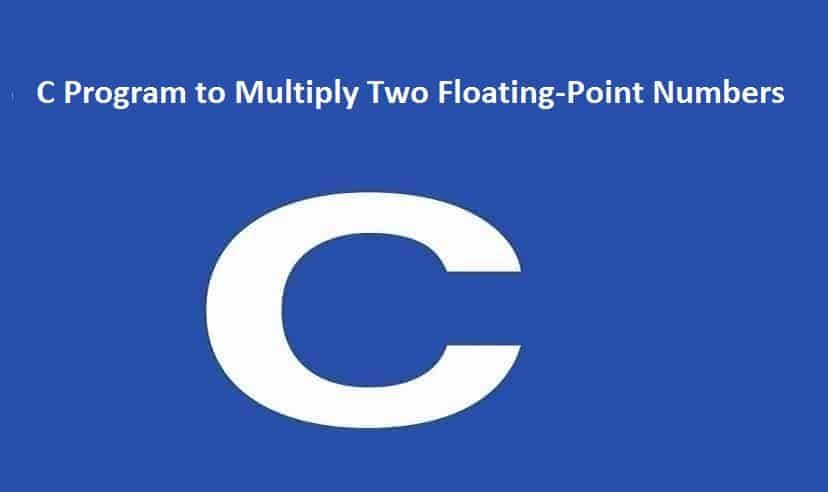## C Program to Multiply Two Floating-Point NumbersC Program to Multiply Two Floating-Point Numbers In this example, the product of two floating-point numbers entered by the user is calculated and printed on the screen. To understand this example, you should have knowledge of the following C programming Read More …